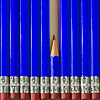# Short Problems for Practice and Revision

The links below take you to a selection of short problems based on UKMT junior and intermediate mathematical challenge questions. You may wish to use them for practice, revision or a mathematical workout!

Longer NRICH problems can be found on the Topics in Secondary Mathematics page.### Number - Short Problems

A collection of short Stage 3 and 4 problems on number.### Algebra - Short Problems

A collection of short Stage 3 and 4 problems on algebra.### Geometry and Measure - Short Problems

A collection of short Stage 3 and 4 problems on geometry and measure.### Handling Data - Short Problems

A collection of short Stage 3 and 4 problems on handling data.### Working Mathematically - Short Problems

A collection of short Stage 3 and 4 problems on Working Mathematically.